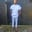Related Tags

d programming
communitycreator

# What is an auto function in D programming?Chinweuba Elijah Azubuike

### What is a function?

Functions are code blocks that carry out specific executions in an application. This code block can be called more than once during the application’s runtime.

### What is the Auto function?

Auto functions are functions without restrictions to their return value type. Let’s see an example.

### Example

import std.stdio;
auto multiply(int val1, double val2) {
double output = val1 * val2;
return output;
}

void main() {
int x = 1;
double y = 2.5;

writeln("multiply(x,y) = ", multiply(x, y));
}
Example of Auto function

### Code explanation

In the code example above we are performing a simple multiplication operation to demonstrate the auto function.

• Line 2: We define our multiply function, notice the auto keyword used while declaring the function. We also passed in parameters that we use in the function.

• Line 3: We define our variable output as a data type of double.

• Line 4: We return the created variable even though it is a double data type.

• Lines 8–9: We define and assign values to our variables x and y.

• Line 11: We print out the result by calling the multiply function inside of the writeln() method.

RELATED TAGS

d programming
communitycreator

CONTRIBUTORChinweuba Elijah Azubuike
RELATED COURSES

View all Courses

Keep Exploring

Learn in-demand tech skills in half the time